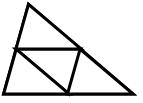favorite We need a little bit of your help to keep things running, click on this banner to learn more
Problems

# TrianglesMike likes to draw triangles, but he does it in an unusual way. First he draws an arbitrary triangle, then divides each side into n equal parts and draws through the partition points the lines parallel to sides of triangle. As a result he gets a number of triangles equal to each other. Help Mike to find the maximum number of identical triangles in his final picture.

#### Input

One integer n (0 < n < 2 * 109).

#### Output

The maximum number of triangles equal to each other.

Time limit 1 second
Memory limit 128 MiB
Input example #1
2

Output example #1
4